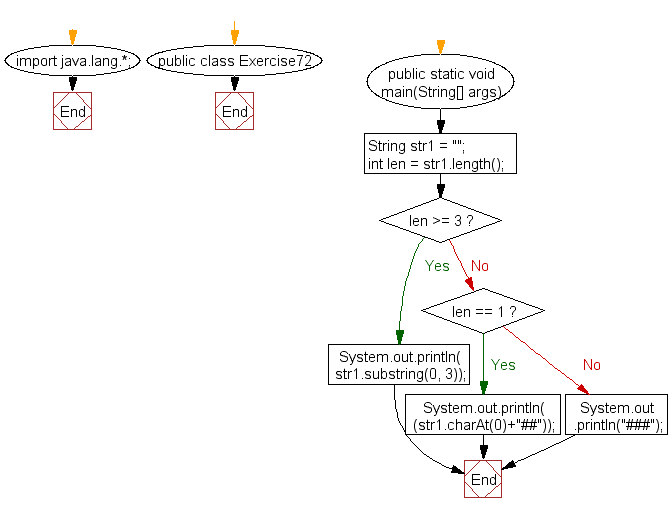﻿ Java: New string taking first three characters from a string# Java Exercises: Create a new string taking first three characters from a given string

## Java Basic: Exercise-72 with Solution

Write a Java program to create a new string taking first three characters from a given string. If the length of the given string is less than 3 use "#" as substitute characters.

Test Data: str1 = "Python"
str2 = " "

Sample Solution:

Java Code:

``````import java.lang.*;
public class Exercise72 {
public static void main(String[] args)
{
String str1 = "";
int len = str1.length();
if(len >= 3)
System.out.println( str1.substring(0, 3));
else if(len == 1)
System.out.println( (str1.charAt(0)+"##"));
else
System.out.println("###");
}
}

```
```

Sample Output:

```###
```

Pictorial Presentation:Flowchart:Java Code Editor:

What is the difficulty level of this exercise?

Test your Programming skills with w3resource's quiz.

﻿

## Java: Tips of the Day

countOccurrences

Counts the occurrences of a value in an array.

Use Arrays.stream().filter().count() to count total number of values that equals the specified value.

```public static long countOccurrences(int[] numbers, int value) {
return Arrays.stream(numbers)
.filter(number -> number == value)
.count();
}
```

Ref: https://bit.ly/3kCAgLb